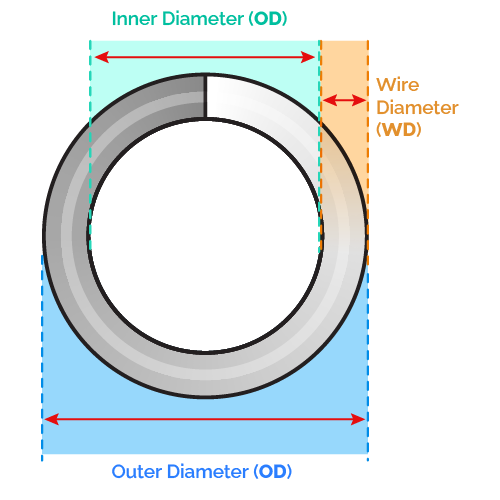# Spring Rate Calculator

Created by Luciano Miño
Reviewed by Steven Wooding
Last updated: Jun 05, 2023

Our spring rate calculator can easily derive the spring rate (spring constant) for any spring, given some of its properties.

In this short text, we will cover the following:

• What is the spring rate or spring stiffness?;
• How to calculate spring rate;
• Different ways to find the spring rate; and

## What is the spring rate?

Spring rate, spring stiffness, or spring constant, refers to the force per unit length needed to stretch or compress a spring. Its units are N/m (newton per meter), or lbf/in (pounds-force per inch).

We can summarize the definition above with the following spring rate formula:

$k = \frac{F}{\delta}$

where:

• $k$ — Spring rate;
• $F$ — Axial longitudinal force applied on the spring; and
• $\delta$ — Displacement produced by the force.

Therefore, we can find the spring rate if we measure both the force and the displacement it produces on the spring.

Keep in mind that the spring rate is a property of the spring rather than a property of its material. For further reading, refer to our Young's modulus calculator.

In our spring rate calculator, however, we will focus on another way of finding the spring rate using only its extensive and intensive properties.

## How do you calculate spring rate?

To calculate the spring rate, we can use the spring rate formula:

1. Write down the material's shear modulus (G).

2. Measure the outer diameter (OD) and the diameter (d) of the wire.

3. Count the number of active coils (n).

4. Replace these parameters in the spring rate formula:

s = G × d4 / (8 × (OD - d)3 × n).In this formula, we didn't submit the spring to external forces. By measuring its physical dimensions and knowing the shear modulus of the material, we obtained the resulting spring rate.

## What are active coils?

In the spring rate formula, the n value refers only to active coils. These are the coils that contribute to the compression/extension of the spring. Depending on how the ends of the spring look, there could be none or up to four coils that don't do any work.

Here's the summary:

• Closed and squared / closed and ground ends: these springs have two unused coils;
• Double closed ends: four unused coils; and
• Open ends: all coils are active.

## FAQ

### Is spring stiffness the same as spring constant?

Yes. Spring stiffness and spring constant reference the same thing: a measure of how much force is required to compress or stretch said spring. The spring constant k is the proportionality factor between this displacement and the force.

### How much force is needed to stretch a 10 N/m spring 0.5 m?

5 newtons (N) or 1.124 pounds-force (lbf). The formula for the absolute value of the strength exerted by a spring is:

F = k·x

where k is the spring rate and x is the displacement. Therefore:

10 N/m × 0.5 m = 5 N

Luciano Miño
Shear modulus (G)
psi
Wire diameter
in
External diameter
in
Internal diameter
in
Spring ends
Open
Active coils
coils
Total coils
Spring rate
lbf/in
People also viewed…

### Circumference

Use this free circumference calculator to find the area, circumference and diameter of a circle.

### Hoop stress

Determine the stresses in thin walled shells (spheres and cylinders) using the hoop stress calculator.

### Sunbathing

Do you always remember to put on sunscreen before going outside? Are you sure that you use enough? The Sunbathing Calculator ☀ will tell you when's the time to go back under an umbrella not to suffer from a sunburn!

### Temperature at altitude

Find the temperature above Earth's surface at any altitude with our temperature at altitude calculator!